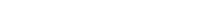# Math riddles Level 28 Answer with Hints and solutionsMath riddles game level 28 Answer with solution android game developed by Black games. Scroll Below to find details.

Math Riddles tests your IQ with mathematical puzzles. Challenge yourself with different levels of math puzzles and stretch the limits of your intelligence. Every IQ game is prepared with an approach of an IQ test. Improve your mathematics, challenge your brain with this game. There are many hard levels which you may not have solved then you can take help from here.

Math app level 28:

4,2 =36

3,7=100

5,4=81

1,6=?Solution: the square of the sum of the two given numbers

there is any Doubt/discrepancy then please let me know in comments or you are unable to understand solution then I will surely help you.

### One thought on “Math Riddles App Level 28 Solution Android”

•July 12, 2019 at 7:05 pm